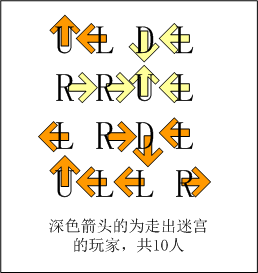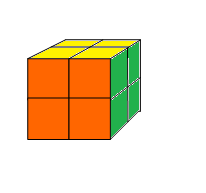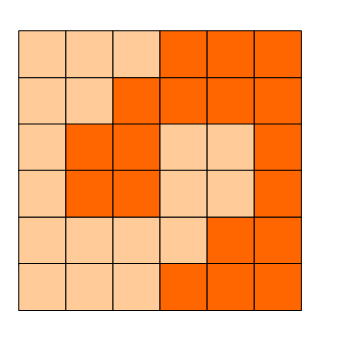signed

QiShunwang

“诚信为本、客户至上”

# 第八届蓝桥杯个人赛省赛详解（Java-A组）

2020/8/20 13:43:59   来源：

1-懒人走迷宫

2-九数算式

3-魔方问题

4-方块分割

5-有限个数字母组合

6-字符串匹配的最大长度

7-正则问题

8-包子凑数

9-分巧克力

10-油漆面积

## 1-懒人走迷宫X星球的一处迷宫游乐场建在某个小山坡上。

L表示走到左边的房间，
R表示走到右边的房间，
U表示走到上坡方向的房间，
D表示走到下坡方向的房间。

X星球的居民有点懒，不愿意费力思考。

------------
UDDLUULRUL
UURLLLRRRU
RRUURLDLRD
RUDDDDUUUU
URUDLLRRUU
DURLRLDLRL
ULLURLLRDU
RDLULLRDDD
UUDDUDUDLL
ULRDLUURRR
------------

``````public class Main {
public static char map[][] = new char;
// 标记走过的路径
static int[][] tag = new int;
static String str = "UDDLUULRULUURLLLRRRURRUURLDLRDRUDDDDUUUUURUDLLRRUUDURLRLDLRLULLURLLRDURDLULLRDDDUUDDUDUDLLULRDLUURRR";
public static int count = 0;

static {
char[] chars = str.toCharArray();
for (int i = 0; i < 10; i++) {
for (int j = 0; j < 10; j++) {
map[i][j] = chars[i * 10 + j];
}
}
}

public static void main(String[] args) {
// 从没个人的角度出发，开始走迷宫
for (int i = 0; i < 10; i++) {
for (int j = 0; j < 10; j++) {
cleanTag();
run(i, j);
cleanTag();
}
}
System.out.println(count);
}

// 数组归零
public static void cleanTag() {
for (int i = 0; i < 10; i++) {
for (int j = 0; j < 10; j++) {
tag[i][j] = 0;
}
}
}

public static void run(int i, int j) {
// 如果当前在界外，说明可以走出迷宫
if (i < 0 || i > 9 || j < 0 || j > 9) {
count++;
return;
} else {
// 如果这一步曾经走过，那意味着走不出去了
if (tag[i][j] == 1) {
return;
} else {
tag[i][j] = 1;
}
// 获取下一步的位置
int nextX = getNextX(i, j);
int nextY = getNextY(i, j);
run(nextX, nextY);
}
}

// 获取下一步横坐标
public static int getNextX(int i, int j) {
char c = map[i][j];
if (c == 'U') {
return i - 1;
}
if (c == 'D') {
return i + 1;
}
if (c == 'L') {
return i;
}
if (c == 'R') {
return i;
}
return i;
}

// 获取下一步纵坐标
public static int getNextY(int i, int j) {
char c = map[i][j];
if (c == 'U') {
return j;
}
if (c == 'D') {
return j;
}
if (c == 'L') {
return j - 1;
}
if (c == 'R') {
return j + 1;
}
return j;
}
}
``````

## 2-九数算式

9213 x 85674 = 789314562

1. 总数目包含题目给出的那个示例。
2. 乘数和被乘数交换后作为同一方案来看待。

分析：

``````public class Main {
static char[] arr = new char[] { '0', '1', '2', '3', '4', '5', '6', '7','8', '9' };
// 每种可能的结果输出数组
static char[] result = new char[arr.length];
// 标记数组,记录数字是否被用过(1为用过）
static int[] tagArray = new int[arr.length];
static int count = 0;

public static void main(String[] args) {
dfs(0);
System.out.println(count / 2);
}

public static void dfs(int layer) {
if (layer >= arr.length) {
if (check(result)) {
count++;
}
} else {
for (int i = 0; i < arr.length; i++) {
if (tagArray[i] == 0) {
tagArray[i] = 1;
result[layer] = arr[i];
dfs(layer + 1);
tagArray[i] = 0;
}
}
}
}

public static boolean check(char[] arr) {
String string = new String(arr);
// 此处需注意，若分割点在第一个位置，则会分为两个字符串，若在最后一个位置，则只会分为一个字符串
String[] strings = string.split("0");
if (strings.length == 2 && !strings.equals("")
&& !strings.equals("")) {
long a = Long.parseLong(strings);
long b = Long.parseLong(strings);
long c = a * b;
return check(c);
}
return false;
}

public static boolean check(Long a) {
String string = a.toString();
for (int i = 1; i < arr.length; i++) {
if (!string.contains(new Character(arr[i]).toString())) {
return false;
}
}
return true;
}
}
``````

## 3-魔方问题## 4-方块分割6x6的方格，沿着格子的边线剪开成两部分。

分析：

``````public class Main {
// 地图数组（0代表未走过的点，1代表走过的点）
int[][] map = new int;
// 方向数组(上下左右）
int[][] direction = { { -1, 0 }, { 1, 0 }, { 0, -1 }, { 0, 1 } };
static int count = 0;

public void dfs(int x, int y) {
// 如果走到边界了，则完成本次切割
if (x <= 0 || x >= 6 || y <= 0 || y >= 6) {
count++;
return;
} else {
// 如何还有可以走的空间，尝试四个方向
for (int i = 0; i < 4; i++) {
// 获取该方向下一步的坐标
int nextX = x + direction[i];
int nextY = y + direction[i];
// 如果该坐标未走过，且该点关于中心对称的点也未走过，则可以走此步
if (map[nextX][nextY] != 1 && map[6 - nextX][6 - nextY] != 1) {
map[nextX][nextY] = 1;
map[6 - nextX][6 - nextY] = 1;
dfs(nextY, nextX);
map[6 - nextX][6 - nextY] = 0;
map[nextX][nextY] = 0;
}
}
}
}

public Main() {
map = 1;
dfs(3, 3);
// 有四级重复
System.out.println(count / 4);
}

public static void main(String[] args) {
new Main();
}
}
``````

## 5-有限个数字母组合

public class A
{
// a个A，b个B，c个C 字母，能组成多少个不同的长度为n的串。
static int f(int a, int b, int c, int n)
{
if(a<0 || b<0 || c<0) return 0;
if(n==0) return 1;

return ________________________________;  //填空
}

public static void main(String[] args)
{
System.out.println(f(1,1,1,2));
System.out.println(f(1,2,3,3));
}
}

6

19

``````public class Main {
// a个A，b个B，c个C 字母，能组成多少个不同的长度为n的串。
static int f(int a, int b, int c, int n) {
if (a < 0 || b < 0 || c < 0)
return 0;
if (n == 0)
return 1;

return f(a - 1, b, c, n - 1) + f(a, b - 1, c, n - 1)
+ f(a, b, c - 1, n - 1);
}

public static void main(String[] args) {
System.out.println(f(1, 1, 1, 2));
System.out.println(f(1, 1, 1, 1));
}
}
``````

## 6-字符串匹配的最大长度

public class Main
{
static int f(String s1, String s2)
{
char[] c1 = s1.toCharArray();
char[] c2 = s2.toCharArray();

int[][] a = new int[c1.length+1][c2.length+1];

int max = 0;
for(int i=1; i<a.length; i++){
for(int j=1; j<a[i].length; j++){
if(c1[i-1]==c2[j-1]) {
a[i][j] = __________________;  //填空
if(a[i][j] > max) max = a[i][j];
}
}
}

return max;
}

public static void main(String[] args){
System.out.println(n);
}
}

分析：

``````public class Main {
static int f(String s1, String s2) {
char[] c1 = s1.toCharArray();
char[] c2 = s2.toCharArray();

int[][] a = new int[c1.length + 1][c2.length + 1];

int max = 0;
for (int i = 1; i < a.length; i++) {
for (int j = 1; j < a[i].length; j++) {
if (c1[i - 1] == c2[j - 1]) {
a[i][j] = a[i - 1][j - 1] + 1;
if (a[i][j] > max)
max = a[i][j];
}
}
}

return max;
}

public static void main(String[] args) {
System.out.println(n);
}
}
``````

## 7-正则问题

----

----

((xx|xxx)x|(x|xx))xx

6

CPU消耗  < 1000ms

分析：

``````import java.util.Scanner;
import java.util.regex.Matcher;
import java.util.regex.Pattern;

public class Main {

public static void main(String[] args) {
Scanner scanner = new Scanner(System.in);
String input = scanner.nextLine();
input = input.trim();
System.out.println(method(input).length());
scanner.close();
}

public static String method(String string) {
if (check(string)) {
return findMax(string);
}
String regex = "[(]x*[|]x*[)]";
Pattern pattern = Pattern.compile(regex);
Matcher matcher = pattern.matcher(string);
while (matcher.find()) {
String str = matcher.group();
String str2 = findMax(str);
str = str.replaceAll("[(]", "[(]");
str = str.replaceAll("[|]", "[|]");
str = str.replaceAll("[)]", "[)]");
string = string.replaceAll(str, str2);
}
return method(string);
}

// (xx|xxxxx) 转化为 xxxx
public static String findMax(String string) {
if (!string.contains("|"))
return string;
string = string.replaceAll("[(]|[)]", "");
String[] strings = string.split("[|]");
if (strings.length() >= strings.length()) {
return strings;
} else {
return strings;
}
}

// 检查字符串是否含有"|"和"(" ")"
public static boolean check(String string) {
return !string.contains("(") && !string.contains(")");
}
}
``````

## 8-包子凑数

----

----

2
4
5

6

2
4
6

INF

CPU消耗  < 1000ms

分析：

“ 如果两个数字不互素，则它们无法组成的数字有无限个，如果两个数互素，则它们无法组成的数字为有限个  ”

``````import java.util.Scanner;

public class Main {
static int dp[] = new int;

public static void main(String[] args) {
Scanner scanner = new Scanner(System.in);
int a[] = new int;
int n = 0, i, j, res = 0, mark = 0;
n = scanner.nextInt();
while (true) {
//获取数字数组
for (i = 1; i <= n; i++) {
a[i] = scanner.nextInt();
}
//找出这些数字中有没有互质的
for (i = 1; i <= n; i++) {
for (j = 1; j <= n; j++) {
if (gcd(a[i], a[j])) {
mark = 1;
break;
}
}
if (mark == 1)
break;
}
//无互质则由无限个拼凑不出来的数
if (mark != 1) {
System.out.println("INF");
continue;
}

//动态规划求出范围内的每个数，能否被组成
dp = 1;
for (i = 1; i <= n; i++)
for (j = 1; j < 10000; j++) {
if (a[i] > j)
continue;
if (dp[j - a[i]] == 1)
dp[j] = 1;
}

for (i = 0; i < 10000; i++) {
if (dp[i] != 1)
res++;
}

System.out.println(res);
}

}

//使用辗转相除法(欧几里得公式）验证两个数是否互素（最公约数为1）
public static boolean gcd(int x, int y) {
int t;
while (y > 0) {
t = x % y;
x = y;
y = t;
}
if (x == 1)
return true;
return false;
}
}
``````

## 9-分巧克力

儿童节那天有K位小朋友到小明家做客。小明拿出了珍藏的巧克力招待小朋友们。
小明一共有N块巧克力，其中第i块是Hi x Wi的方格组成的长方形。

为了公平起见，小明需要从这 N 块巧克力中切出K块巧克力分给小朋友们。切出的巧克力需要满足：

1. 形状是正方形，边长是整数
2. 大小相同

2 10
6 5
5 6

2

CPU消耗  < 1000ms

``````import java.util.Scanner;

public class Main {
public static void main(String[] args) {
Scanner scanner = new Scanner(System.in);
// 巧克力数量
int num = scanner.nextInt();
// 小朋友人数
int a = scanner.nextInt();
int[][] arr = new int[num];
// 巧克力数组
for (int i = 0; i < num; i++) {
arr[i] = scanner.nextInt();
arr[i] = scanner.nextInt();
}
// 逐次增加分割的块边长
for (int i = 1; i < 100000; i++) {
// 可以分割的块数
int m = splitAll(arr, i);
if (m >= a)
continue;
if (m < a) {
System.out.println(i - 1);
break;
}
}

}

/**
* 求出所有巧克力能将分多少块
*/
public static int splitAll(int[][] arr, int sideLenght) {
int count = 0;
for (int i = 0; i < arr.length; i++) {
count = count + split(arr[i], arr[i], sideLenght);
}
return count;
}

/**
* 求出一块巧克力能将分多少块
*/
public static int split(int height, int width, int sideLenght) {
int x = height / sideLenght;
int y = width / sideLenght;
return x * y;
}
}
``````

## 10-油漆面积

X星球的一批考古机器人正在一片废墟上考古。

(0<= x1,y1,x2,y2 <=10000)

3
1 5 10 10
3 1 20 20
2 7 15 17

340

3
5 2 10 6
2 7 12 10
8 1 15 15

128

CPU消耗  < 2000ms

``````import java.util.Scanner;

public class Main {
public static void main(String[] args) {
boolean[][] map = new boolean;
Scanner scanner = new Scanner(System.in);
int num = scanner.nextInt();
for (int i = 0; i < num; i++) {
int x1 = scanner.nextInt();
int y1 = scanner.nextInt();
int x2 = scanner.nextInt();
int y2 = scanner.nextInt();
for (int x = x1; x < x2; x++) {
for (int y = y1; y < y2; y++) {
map[x][y] = true;
}
}
}
int count = 0;
for (int i = 0; i < 10000; i++) {
for (int j = 0; j < 10000; j++) {
if (map[i][j]) {
count++;
}
}
}
System.out.println(count);

}

}
``````